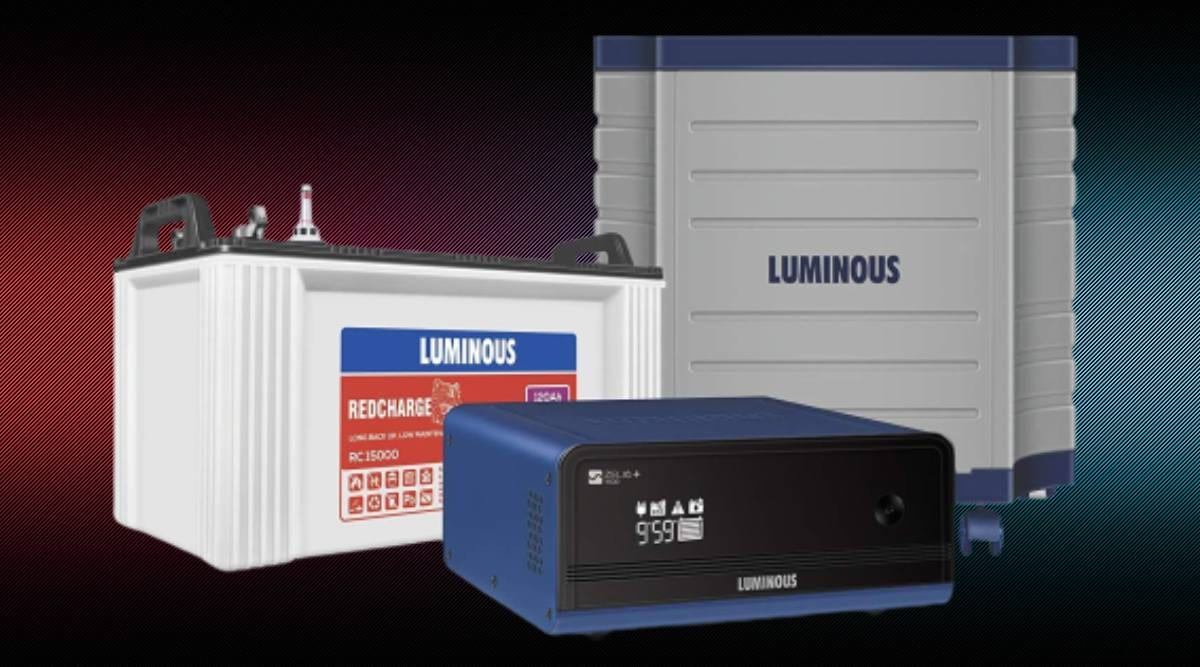# How to choose the right inverter and battery for your home

Peak summers are almost here and if you’re like me, summers could be synonymous with power cuts in your locality. In some areas, power cuts can be a relatively short affair, with outages ranging from 15 minutes to 30 minutes. However, some areas will witness power cuts of multiple hours on a daily basis. These scenarios can be troublesome if you study or work from home, not to mention appliances fans and inductions don’t when you face power cuts.

The only real solution left is to get an inverter and battery setup for your home. Unfortunately, while getting an inverter has never been easier thanks to eCommerce and doorstep delivery, choosing one can be a little challenging for some. In fact if you don’t exactly know what you’re looking for, you may end up paying more for a product that is actually less useful for you.

To avoid such mishaps, we will today be looking at how to choose an inverter for yourself, in a few simple steps.

## How to select an inverter and battery

Note: The basic rules are that the inverter you select should be determined by the number of appliances you want to use during power outages, while the battery you select will depend on how long you want the power output for.

We will calculate our needs in a few easy steps.

Planning

Before you get an inverter, half of the job is planning for the right one. To do that you need to make a list of appliances that will be connected to the inverter. This will help you understand what the total wattage that you need is.

For instance, let’s say I need my inverter connected to only one 25W fan, one 60W TV and two 40W LED bulbs. The total wattage I need would be 25+60+40+40 which is 165W. You can then keep another 100W of buffer if you think you could be adding more plug points or appliances to the inverter in the future. Let’s say the total wattage along with this buffer amount is 265W.

Choosing an inverter

To choose the right inverter, you need to find your V/A rating (Volt/Ampere). There are a few steps to do this.

Your required Volt Ampere rating is essentially your required power (in Watts) divided by the power factor of the inverter you are considering.

The required power is the number of Watts that we calculated in the previous step. The power factor of an inverter is basically the efficiency at which the inverter operates.

An inverter will never be able to operate at 100 per cent efficiency. It will instead, likely operate at between 50-80 per cent efficiency. If its efficiency is 70 per cent, its power factor will be 0.7, if its 80 per cent, the power factor will be 0.8 and so on. Let’s assume the inverters we are looking at all have a power factor of 0.7.

Once you have the power factor, calculating your Volt Ampere rating is easy. Simply divide your required wattage by the power factor of the inverter.

In our case, we will be dividing 265W by 0.7, which is equal to about 378VA. You can now pick any inverter that has a higher Volt Ampere rating than 378VA. Try not to pick a number too high though, as that will simply be a waste of money.

Choosing a battery

You’ve chosen an inverter, but you still need a battery that holds the power in it for when the power cut hits. Here’s how to choose the right battery for yourself.

Battery capacities are measured in Ampere Hours (Ah). To determine what Ah reading you need on a battery, you must do a couple more basic calculations.

Remember this formula –

Battery capacity = {Power requirement (Watts) multiplied by Num of hours} divided by Battery Voltage (Volts).

Don’t spend more on the wrong inverter, check out how to select the right one here. (Image Source: Amazon India)

Battery voltage is usually 12V. Hence, our needed battery capacity for keeping our appliances up for say, three hours would be…

{Power requirement (265W) multiplied by Num of hours (3 hours)} divided by Battery Voltage (12V)

Which is (265×3)/12 = 66.25Ah. So any battery capacity above 66Ah would be enough for you. Although do note that you can always go for a higher battery capacity for longer power times. That’s it. you have now calculated what inverter and battery you need.